Example Questions

← Previous 1

Example Question #1 : Measurement And Data

What is the length of a rectangular room with a perimeter of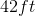and a width of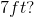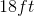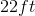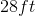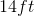Explanation: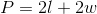We have the perimeter and the width, so we can plug those values into our equation and solve for our unknown.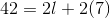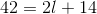Subtractfrom both sides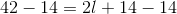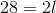Divideby both sides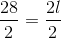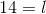Example Question #2 : Measurement And Data

David wants to put a fence around his backyard. How much fencing will he need if his backyard is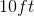by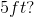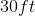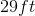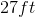Explanation:

The fence is going around the backyard, so this is a perimeter problem.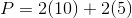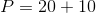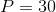Example Question #3 : Measurement And Data

If we wanted to make this angle into a straight line, how many more degrees would we need to add?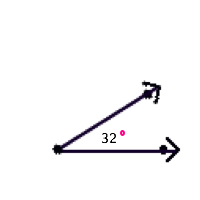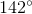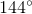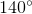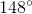Explanation:

A straight line has a measurement of. We can subtract to find how much more we'd need to have a straight line.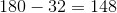Example Question #1 : Map 4th Grade Math

The triangle below can be classified as what type of triangle?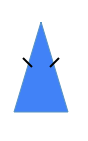Equilateral triangle

Scalene triangle

Right triangle

Isosceles triangle

Isosceles triangle

Explanation:

This is an isosceles triangle because an isosceles triangle has two equal sides.

This triangle is a not scalene triangle because a scalene triangle has side lengths of all different lengths.

This is not an equilateral triangle because an equilateral triangle has to have all sides equal in length.

This is not a right triangle because a right triangle has to have a right angle, an angle that measures.

Example Question #2 : Map 4th Grade Math

Does the black line in the figure represent a line of symmetry?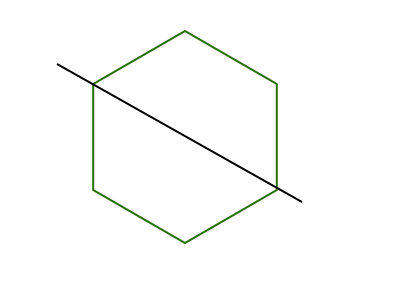Neither choice is correct

Both choices are correct

No

Yes

Yes

Explanation:

When you are trying to decide if a line represents a line of symmetry you can think about folding the figure across that line. Once you make the fold, do all of the parts line up on top?

If yes, then the line represents a line of symmetry.

If no, then the line does not represent a line of symmetry.This line represents a line of symmetry because if you fold on the black line, then one side would lay on top of the other and the parts would match up.

Example Question #3 : Map 4th Grade Math

The triangle below can be classified as what type of triangle?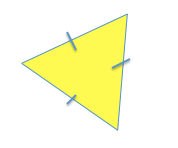Scalene triangle

Right triangle

Equilateral triangle

Isosceles triangle

Equilateral triangle

Explanation:

This is an equilateral triangle because the sides are all equal, and an equilateral triangle has to have all sides equal in length.

This triangle is not a scalene triangle because all of the side lengths are not different lengths.

This is not an isosceles triangle because an isosceles triangle has only two equal sides.

This is not a right triangle because a right triangle has to have a right angle, an angle that measuresExample Question #1 : Numbers And Operations

Select the decimal that is equivalent to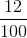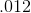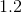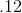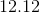Explanation:is twelve hundredths.is twelve hundredths. When we say a decimal, we say the number and add the place-value of the last digit.

Example Question #1 : Numbers And Operations

Fill in the blank with the missing fraction.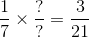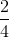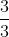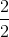Explanation:

In this problem we are making equivalent fractions. In order to make equivalent fractions you have to multiply the numerator and the denominator by the same number.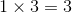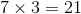Example Question #3 : Numbers And Operations

Select the symbol to correctly fill in the blank below.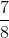__________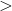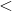Explanation:

To compare fractions, we need to first make common denominators.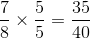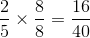Now that we have common denominators, we can compare numerators. The fraction with the bigger numerator has the greater value.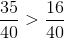Example Question #1 : Operations And Algebraic Thinking

Justin hasshelves in his room. Each shelf holdstoys. If Justin hastoys, how many toys don't fit on the shelves?Explanation:

To solve this problem, we first need to find out how many of Justin's toys will fit on the shelves. If we haveshelves, andtoys fit on each shelf, we can multiply those two numbers together to find out the total number of toys that will fit. Let's letrepresent the number of toys that will fit.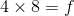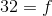Justin hastoys, so to find out how many don't fit on the shelves we need to know what is left over, so we subtract. Let's letrepresent the number of toys left over.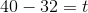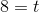← Previous 1